What’s a sphere?

Geometrically, a sphere is the locus of points equidistant from a given point (which we call the ‘center’. Algebraically, it is the solution set to the equationwhere d is the number of dimensions the sphere lives in, and r is some positive constant which we call the radius.

In one dimension, a 0-sphere is just two points, one on each side of the ‘center’. In two dimensions we call a 1-sphere a circle. The words sphere itself is typically reserved for a 2-sphere. Higher dimensional (d-1)-spheres are typically just referred to as hyperspheres. Why d-1? Think of a circle in two dimensions… how many dimensions does the circle actually have? You can only slide along the circle either forward or backward, hence it is one dimensional. A moment thinking about a sphere in three dimensions will get you to the same conclusion

So, whats the surface area of one of these hyperspheres? We could do some nasty area element calculation from the algebraic constrain above, but that would make everyone cry (except Russians…. Russians do not need fancy techniques when they can do something with brute force). Instead, let’s integrate an isotropic multivariate Gaussian with vanishing covariance two ways. A what now? Consider the integral: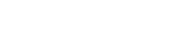where the covariance matrix is diagonal with equal entries (σ²). Because of the pleasant structure of the covariance, we can break this integral up into d Gaussians and integrate:Yay! Well… not yet, stop being so excited.

To connect this to hyperspheres we’re going to change to polar coordinates. The meassure will then be over all radii and solid angles:where we have used the definition of the Euler Gamma Function. Try doing the integral yourself! We can now equate these two results and solve for the total solid angle of a hypersphere in d dimensions, and then get the surface area of a hypersphere:We can plot this as a function of dimension (because of our know how with the Gamma function, we can even look at fractal dimensions…):The function maximizes at d=7.25… dimensions. That’s weird. WHAT DOES IT MEAN?!?!?!?!?

Well, the total solid angle of a space can be thought of mechanically as how much you would have to move your head around to survey everything in every direction. Clearly in 1d you just have to look ahead of you and behind you. In 2d you have to pull an Exorcist move, and spin your head 360 degrees. In 3d you have to add to that and look above and below you. As you keep increasing the number of dimensions, the amount of neck movement keeps getting worse. Until you hit 7 dimensions. Then as you increase the number of dimensions, you realize you have to move your head less and less. In fact, by the time you’re in 18 dimensions, you have to move your head less than in 1 dimension, to see everything. Higher dimensions are weird.

Ok, what about the volume of hyperspheres? That’s pretty easy. We just integrate from a radius of 0 to some final radius to get: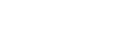This too is interesting. For a fixed radius, The volume maximizes as d = 5.25… dimensions. Higher dimensional unit-spheres just don’t take up that much space. Finally we can also quickly calculate the surface area to volume ratio. This turns out to be just d/r. As expected, the larger hyperspheres get, the more volume to surface area they have.

This scaling is very important, and the main reason Life uses cells as its fundamental unit. The energy usage of a cell is proportional to its volume, whereas its energy absorption is proportional to its surface area. If this ratio of absorption to usage is greater than one, then the cell is making excess energy and can grow in size. Eventually, however, because this ratio is proportional to the surface area to volume ratio, it will become less than 1 as the radius increases, and the cell will not be able to support further growth. At this point it is more efficient to split into two cells and CELLS ARE EVERYWHERE.

Interestingly, due to the scaling with dimension, we would expect cells that evolve in universes with more spatial dimensions to be larger. Assuming energy usage and absorption don’t change in higher dimensions, we would not expect multi-cellular life to ever evolve in worlds where the number of spatial dimensions is incredibly high. Someone really should make a B-movie entitled: Attack of the Amoeba of a Billion Dimensions!!!!

# Differential EntropyShannon entropy is defined over a set of discrete events. One might wonder what happens when we try to generalize Shannon entropy to a continuum of events. It turns out that one will run into problems stemming from the infinite degrees of freedom in the continuum. Nonetheless, it will still be possible to define a modified form of entropy. Let’s see how.

Consider an experiment where we can measure a continuum of possible values ( the position of a particle, for example). The first thing we will do is divide the domain of possible outcomes into a discrete set. The probability that an outcome is measured to within Δx of x requires the introduction of a probability density function, ρ: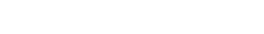where we have chosen Δx to be small enough so that the probability density is approximately constant within that range. We can now write down the standard formula for Shannon entropy: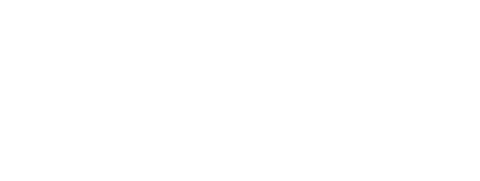The first term looks nice, but the second term diverges. If we think of entropy as hidden information, then this result is quite obvious. It would take an infinite number of questions on average to guess a randomly chosen real number.

The differential entropy is the defined by the first term in the above:Though this looks very similar to Shannon entropy, we mustn’t jump the gun and apply the intuition we learned there here.

Unlike entropy, differential entropy is not positive definite. One can quickly see this by considering the entropy of a Gaussian distribution(check!):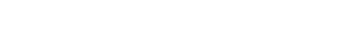If the variance of the distribution gets too small, the differential entropy becomes negative. Thus a delta function has infinite negative differential entropy (take the limit of the Gaussian). This is in stark contrast with the discrete case where a fully selective probability distribution has vanishing entropy.

Because of this we can no longer use our intuition in thinking about differential entropy as hidden information. It does not represent the number of questions that one must ask in order to reveal the hidden information in an experiment. Fortunately we will see that continuum generalizations of other information measures won’t suffer from the same issues as differential entropy.

# Shannon EntropyIn this post we will describe the information measure known as Shannon Entropy. What is it? What does it mean? How is it used?

As a bit of history, Shannon developed this measure in his revolutionary paper A Mathematical Theory of Communication in 1948. This paper was groundbreaking because it single-handedly  gave birth to information theory. Since its publication, it’s been cited nearly 100,000 times, which is 6 times as much as Einstein’s most cited paper written with Podolsky and Rosen. Though modern day technology has hardware rooted in our understanding of Quantum Mechanics, the relaying of information between devices would be unimaginably slow if the software wasn’t grounded by Shannon’s work. Shannon entropy is used to prove two coding theorems (noiseless and noisy), firmly establishing how encoding schemes make modern communication as fast as it is.

So, what is Shannon Entropy?

Think of it as the amount of hidden information contained in an experiment. Yeah, I know, what the hell does that mean? Bare with me.

Hidden information is hidden, because it must be revealed. How do we reveal information that is hidden? Well, how do I find out what your age is if I don’t know it? I ask you. Hidden information is revealed by asking questions. The total amount of hidden information is then just the total number of questions that one needs to ask to find out the result of an experiment.

Already we have some problems with this definition.

1. What kind of questions are we talking about? Some questions have 2 answers (are you older than 20?), while others have many more (how old are you?).
2. If I ask the right questions, then I can get the answer quickly, and if I ask the wrong ones it will take me longer. How can you say total number of questions when that number can be high, low, or in between depending on how I ask questions? The total number of questions is not invariant.

Both of these objections are actually not problems at all. For the first question, the number of answers that a question has is referred to as its base, b. It turns out that each question can have a different base, though for ease of computation we will assume that b is fixed. If b=2, the unit for number of questions is called the bit. This is the convention we will use from now on, though other conventions exist. b=10 gives us dits, while b=e (useful for analytical work) gives us nats.

The second objection realizes that there is a subjective component to asking questions. To get around it, the total number of questions (in a particular base) means the minimum over questioning schemes when that questioning scheme is used over all possible realizations of the experiment. An example will help with this one.

Consider  a situation where we have N boxes arrayed in front of us. In one of them there is a marble. Our goal is to reveal the information of which box the marble is in. A naive questioning scheme would be “Is it in box 1?,” “Is it in box 2,” etc. Sometimes we’d get the answer very quickly. Other times it would take us a long time. The expected number of questions we would have to ask is (N+2)(N-1)/2N (check!), which grows asymptotically as O(N). This is a rather inefficient questioning scheme.

A more(most) efficient scheme would consist of subdividing the boxes into groups of 2. You could ask “Is it in this half?” and with each answer reduce the number of possible answers in half. No matter where the marble is, the expected number of questions will be log N, where the logarithm is in base 2. This expectation grows as O(log N) asymptotically, and is in fact the optimal questioning scheme. Hence the experiment of guessing which box a marble is in has hidden information log N.

Now let’s change things up by considering how knowledge affects hidden information. If I asked you to guess the first letter in an English word, you might think in analogy to the last problem and say that there is log 26≈4.7 hidden information in this experiment. It turns out that there is less than that because you know that the letter is in an English word. You’re going to choose letters that are more common, and not the weird ones. On average, you’ll have to ask 4.2 questions because of this prior knowledge.

So, how does all of this translate into mathematics? Shannon found that for an experiment with N possible outcomes that have associates probabilities to each outcome, the hidden information reads:The amazing thing here is that this functional form is the unique solution to several very simple requirements. Probably the most concise statement of these axioms comes int he form given to us by Kinchin in 1957:

1. If we permute the labels of the outcomes, then this must have no effect on hidden information. Labels are extrinsic.
2. If the distribution is uniform, then H should be at a maximum. This says that the uniform distribution hides the most information from us relative to any other distribution over the same outcomes.
3. We can extend the distribution to include more events that have 0 probability. This must have no effect on H.
4. For any two random variables X,Y, the joint hidden information must satisfy the subadditive constraint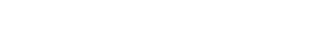The last constraint ensures that the hidden information in X and Y is equal to the hidden information in X plus the hidden information in Y if and only if the two events are independent of one another. A more in depth mathematical description can be found here.

The second of Kinchin’s axioms is interesting. Plugging in a uniform distribution we find H=log N, which is the same result we found in our marble example. This is because we had no other information about the location of the marble: our prior was uniform. What if instead we had complete information about the results of the experiment and knew which box the marble was in. Using an indicator function we find that H=0. There is no hidden information if its all been revealed!

Entropy in physics is typically associated with disorder. In information theory, entropy is synonymous with hidden information. These two ideas, disorder and hidden information, turn out to be related to one another. If a system is disordered, then it takes a lot of questions to pinpoint the state of the system. Imagine a messy room and how long it takes to find all the socks. Now imagine a clean room where all the socks are in the sock drawer. Once you find one sock, you’ve found them all. Sometimes physicists even use the word negentropy to mean information.

Thinking about entropy in this information theoretic fashion can give one a better intuition for what entropy is physically. The connection between statistical physics and information theory lays at the heart of much of modern physics. It is an ongoing field of research that is full of fascinating topics which include black hole thermodynamics, and quantum computing. Hopefully we’ll get to explore these in future posts. Next, however, I want to look at the connection between prior knowledge, or constraints, and hidden information. This will lead us to the principle of maximum entropy.

# 1-d Ising Model: Transfer Matrix MethodIn this post we will develop the transfer matrix method for solving the one dimensional Ising model. Hopefully the post will be pedagogical enough so that beginners can use it as a launching point into their investigations of more interesting systems.

We begin with a one-dimensional grid with$N$ vertices. The state of the$i^{th}$ vertex is denoted$\sigma_i\in \{1,-1\}$. We impose periodic boundary conditions so that$\sigma_{N+1} = \sigma_1$. The Hamiltonian can be written in a symmetric form:The components of the transfer matrix are defined asso that the transfer matrix readsSome of you may now be thinking “why in the world did we decide to do that?” I apologize for the preemptive definition without any form of motivation, but bear with me. All we need to do is look at the partition function for this system to see the utility of the transfer matrix: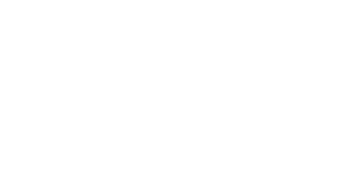Voila. The partition function can be written as the trace of the transfer matrix exponentiated to the power of the number of vertices. Why is recasting the partition function in the language of linear algebra important here?  Well, because we can now use our arsenal of tricks to simplify the trace. If we can diagonalize the transfer matrix,$T = S^{-1}\Lambda S$, then, using the cyclic property of the trace, we can write:where the$\lambda_i$ are the eigenvalues of the transfer matrix, ordered so that$\lambda_1 \ge \lambda_2$. The eigenvalues are the solutions to the characteristic equation, which for a$2\times 2$ matrix reads: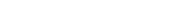where$\text{Det}$ is the determinant. The two solutions are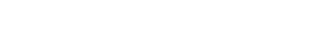Here’s a visualization of both of them. Clearly, the eigenvalue with the positive sign is greater than the other for all values of the coupling and the magnetic field (Can you prove this?). Hence it  plays the role of$\lambda_1=\lambda_+$. In the thermodynamic limit, we have then that:I can’t stress how nice it is that we can derive an expression for the partition function here. For the majority of problems, calculating the partition function is not possible, so this example really is a very special case from which we can gain a lot of insight. We can instantly read off thermodynamic quantities by taking derivatives. In particular we find that the mean magnetization is: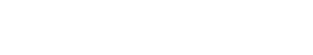Since this drops to 0 when the external field vanishes, we see that there is no spontaneous magnetization in the 1 dimensional Ising model, a fact originally discovered by Ising himself. Unfortunately, Ising then went on to assume that this means that there is no phase transition in the model in any number of dimensions, which is not true. You can read more about the history of how the critical temperature of the phase transition in 2-dimensions was discovered over the course of the three decades that followed Ising’s analysis of the one dimensional model here.

# Entropic Compression and EnglishLet’s say that we want to send a message to a friend. What’s the smallest amount of information we need to send that message? The answer to this question is related to the entropy of the alphabet we use to write the message in. This post will cover exactly how to do that.

First some definitions. A letter is a symbol, which we denote$a_i$, used to write messages. The subscript runs over all the letters in the alphabet being used,$\mathcal A = \{a_i\}_{i=1}^N$. The size of the alphabet we use is$N$ letters long. A message consists of a string of letters,$\mathcal M = a_{i_1}a_{i_2}\cdots a_{i_M}$. The size of the message is$M$ letters. To convey the message we need to store each letter and send it, so the way in which we store letters will determine the amount of storage we need to send the message.

Let’s first think through what we mean by storage. Since the alphabet has$N$ letters, each letter can be stored in either a single N-it, or in$\log N$ bits. Think of the latter as the number of yes/no questions one needs to answer to pinpoint which element of the alphabet a particular letter is (If the logarithm is fractional then we need to round up). Hence the entire message needs$M \log N$ bits to be sent. Is this the best we can do? Obviously no, otherwise this post wouldn’t exist.

The key to compressing the message is to use the entropy of the alphabet. The lower the entropy of the alphabet, the more compression we can achieve. The key is to store the letters of the alphabet so that the most common letters use up less storage (fewer bits). This is at the expense of rare letters using more space. This means that certain messages, when restored under this new scheme, will actually use up MORE storage. However, these messages are rare, and, on average, the majority of messages will take up less storage.

So, how is this new storage scheme accomplished?

First we need to find the probability of appearance of each letter,$p_i = p(a_i)$. This can be done by looking at a corpus of messages that have already been sent to see which letters are used more often than others, or through the use of an epistemic agent that starts off ignorant and observes messages, refining its belief distribution for the alphabet. Once the probabilities are known, the information content in each letter is:The ceiling of this value is the number of bits entropic compression requires to store that particular letter. Hence, each letter can be mapped to a code word that is that many bits long. So what is the average number of bits needed to store a particular letter?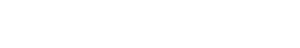Voila! The entropy of the alphabet coincides with the average number of bits needed to store a letter. Since the entropy is maximized for an equiprobable alphabet at$S^{max} = \log N$, this scheme is will use no more space than the original. To see this we consider long messages. The total information needed to transmit them will be$M S[\mathcal A] \leq M\log_2 N$. Now, there is a small caveat here, since we did use the ceiling function, but we won’t worry about that.

So what does this mean for messages sent in English? Assume an alphabet of only 26 letters (don’t distinguish between upper and lower case and no spaces). The average number of bits needed to convey a letter is$\log_2 26\approx 4.70$. But we aren’t writing just any messages, we’re writing messages in English. I downloaded the 11th edition of Encyclopaedia Britannica, which gave me a dataset of over 40,350,587 words. Let’s take some time to discuss it, first starting with letters, and then moving up to clusters of letters and words.

Parsing up all those words into their constituent letters gave me 192,439,482 instances of letters in the English language. How often does each letter occur?As you can see ‘E’ is the winner, and vowels show up pretty often. Q’s and Z’s do not. We can calculate the entropy of English based of of this letter distribution, and find that the average information needed to store a letter is$S[\mathcal A] \approx 4.17$. Hence this scheme can compress messages by about 11%. Now to actually implement this scheme requires figuring out the code words to map each of the letters to. This can be accomplished quite elegantly using a Huffman tree, which also gives entropic compression its standard name: Huffman coding.

It should also be mentioned that this compression scheme also provides the maximum compression that can be accomplished based on the constraint imposed by letter frequencies on which we trained the epistemic agent whose beliefs we’re using. This is because the maximized entropy under the constraint is the most ignorant belief function allowed for: lowering the entropy implies that the epistemic agent is not maximally ignorant. But if the EA is not maximally ignorant beyond the information given to it by the constraint, that means that there must exist some other information (constraint) that it is using to constrain its beliefs. Since, by assumption, the frequency constraint is all the information the EA has to work with, a lower entropy implies a contradiction.

Now, this compression is great, but can we add more constraints from the English language to make it even better? What sort of constraint should we add?

If you are given a word to guess the first few letters might take around 4-5 tries. That’s what the entropy means! You’ll need to ask on average 4.17 questions to get the right letter. However, once you have the first one or two letters you’ll be able to guess the remaining letters pretty quickly. If you see a ‘q’ you’re going to guess ‘u’  is the next letter and you’ll most likely be right. That’s because English has much more structure than just the frequency of letters. Some letters tend to follow other letters more often than others. This following preference can help us construct an even better compression algorithm!

Once again I took the Encyclopaedia Britannica and did an analysis of which letters follow each other. I got the frequencies for all pairs of letters in words, and then calculated the conditional probabilities via Bayes’ Rule: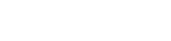The conditional probabilities are referred to as 1-transitions, since they tell you what the probability is of transitioning to a new letter given that you have 1 letter of knowledge. The results are pretty neat:The scale is logarithmic, so the colors go in powers of 2, and the sum across rows is normalized. The difference between red and blue is 16, so red is over 64000(2^16) times as likely as blue. Note the patterns in this plot. Consonants love to be followed by vowels. ‘B’ loves to be followed by ‘Y’ and ‘E’. ‘Q’ is almost always followed by ‘U’. There are some weird ones in there too. Why is ‘Q’ sometimes followed by ‘V’? That’s probably due to the fact that the Encyclopedia’s digital version hasn’t been finished being edited. The original was scanned and turned into letters based on the scanned shapes. In older books (and this one is from 1911) had u’s printed as v’s.

Neat, so now we have transition probabilities. How picky are the transitions for different letters? That can be answered by looking at the entropy of each of these distributionsThe dashed green line is the entropy of the alphabet. Notice that all the transition entropies are lower, meaning that if you know a letter, it takes less guesses to find the next letter. For letters on the right side of the plot, much fewer guesses are needed. The letters on the left side are less picky about followers, and more guesses are needed. We can perform a Huffman encoding on each of these distributions, and further compress our English messages. As long as the decoder has this list of lists of codewords they will be able to decipher what we send them.

So, how much have we gained by looking at next letters?

The answer has to do with the conditional entropy of the joint distribution of two letters. Put plainly, the entropy of two letters minus the entropy of the first letter gives us the average number of questions we need to ask to guess the second letter if we know the first (Translation: (how many questions do you need to guess the pair of letters) – (how many questions you need to guess the first letter)):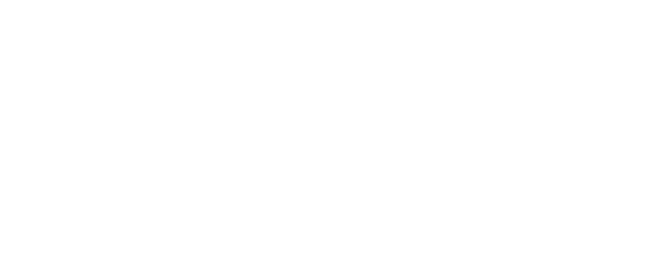Calculating this entropy we get$S[\mathcal A|\mathcal A] \approx 3.29$, or a 30% compression from the original. We’ve almost doubled our compression by considering which letters are more likely to follow which letters.

But why stop there?

Why not look at the probability that a letter follows a pair of letters: the 2-transition probabilities? Or the 3-transition probabilities, etc. Doing this for the data set, I found that English has$S[\mathcal A|\mathcal A\times\mathcal A] \approx 2.82$,$S[\mathcal A|\mathcal A\times\mathcal A\times\mathcal A] \approx 2.03$,$S[\mathcal A|\mathcal A\times\mathcal A\times\mathcal A\times\mathcal A] \approx 1.19$. If we plot the entropies based on the number of constraints we’re using, we find something amazing:The line at an entropy of 1 corresponds to one wrong guess, on average, to get the next letter right. We clearly see a linear trend in the entropies approaching zero. If the trend continues then we expect perfect guessing of what the next letter will be somewhere around 6 priors. So what does this mean about compression? Well, it means we need to spend the most bits (on average) on the first letter of each word in a message, and fewer bits on each consecutive letter in that word. We shouldn’t even send the letters beyond the 7th in long words because they’ll be easily filled in by the decoder(most of the time). Recall that with Huffman encoding we were able to reduce the size of a word in a message to$MH \approx M\times 4.17$ bits. What is the average storage we need now?

To answer that we really need the word distribution of English: How many words of each length appear in the language? There’s a nuance here, in that we are interested in the usage of the language, and not the corpus of words that make up the language. The latter is over all possible unique words, whereas the former includes repetition. We can plot both distributions:This is a cool diagram because it tells us that the distribution of used words pulls the average letter count per word down quite a bit. Treating words as unique entities, the mean word length of English is 8.37 letters per word, and 50% of words have less than 7.46 letters in them. Treating words as non-unique elements of messages the mean word length is 4.78 letters per word, and 50% of words have less than 3.55 letters in them. The distributions are equal at 5.19 letters per word, meaning that English words with more than 5 letters are underused, while words with 5 or less letters are overused.

Since we’re dealing with how English is being used to make messages, we should use the blue distribution to calculate the average entropy of a word. We do this by averaging the entropy needed to store a words of length l over all possible word lengths:The result is pretty amazing.

How amazing?

Consider the original scheme of storing each letter of the alphabet using the same amount of storage. The average word entropy would be about 22.42 bits. With entropic encoding, the entropy we have as a result of the above is 11.44 bits. We’ve achieved a compression of about 50%, which basically allows us to send twice as many messages as before. Pretty neat, huh?

# The Ising Model: A Graph Theoretic IntroductionIn this post I will introduce the Ising model from a graph theoretic point of view, without resorting to a particular graph structure on which the system lives. I will explore more specific systems in a future post.

Consider an undirected graph$G=\langle V,E \rangle$ where$V$ is a set of vertices, and$E$ is a set of edges. A state of the graph,$\sigma_G$, is a mapping of the vertices,$\sigma: V\rightarrow \{-1,1\}: v_i \mapsto \sigma_i$, and the edges,$\sigma: E\rightarrow \{-1,1\}: e_{ij}\mapsto \sigma_{ij} = \sigma_i\sigma_j$, where i and j are the vertices that bound the edge. This definition makes edges that bound vertices of the same state have a state of +1, and edges that bound vertices of opposite states have a state of -1.

Since each vertex can be labeled in one of two ways, and the state of the edges is fully determined by the state of the vertices, there are a total of$2^{|V|}$ possible states for the graph, where$|\cdot|$ is the cardinality operator. We bundle these together and write$\Sigma_G = \{\sigma_G\}$.

We want to compare different states to one another, and the easiest way to do this is to define a Hamiltonian. A Hamiltonian is a function that maps states to real numbers, which we call energies. It consists of a piece that acts on the edges, and a piece that acts on the vertices:where the f’s are coupling functions. In the simplest case we choose$f_0(x)=hx$, and$f_1(x) = -Jx$. In the physics parlance, h is referred to as the magnetic field, and J the coupling. Plugging in the definitions for the state of vertices and edges, the form of the Hamiltonian that we will be examining reads:where the second sum on the first line is over unique pairs of vertices, and on the second line over the neighbors of the ith vertex. The factor of a half comes from overcounting each vertex twice in the complete expression. The reason for the negative sign is that it ranks states that are more homogeneous lower, so we can say that homogeneity decreases energy.

We can make the notion of homogeneity more precise by introducing an order parameter, called the magnetization, describing the average vertex state:Note that this is a number between 1 and -1, inclusive. There are many states that have the same order parameter. So many, in fact, that’s it’s best to talk about the logarithm of the number, which turns out to be (for very large graphs):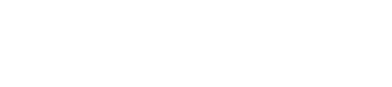Here I used Stirling’s approximation for the factorials in the binomial coefficient. This function is peaked close to 0, especially for large graphs. The majority of the states have little or no magnetization; in fact if we expand around 0 magnetization we see that the states are distributed as a Gaussian:Here I have included the quartic term in the Gaussian, which can be neglected. To see this we plot the distribution below for graphs with 10,100,and 1000 vertices. We see how quickly the distribution becomes sharply peaked at vanishing magnetization:What does this mean?

We imagine that the system has some sort of dynamics: that it can transition from one state to another state. Under no other constraints, the system will soon find itself in a state with little or no magnetization.

For something more interesting to occur, the dynamics of how the system transitions from one state to another must be constrained by more than simply the available volume of phase space. Here is where we finally get to the physics of the Ising model. The constraint we need to incorporate is that the energy of the system is constant, so that we can say what the probability of each state is:$p(\{\sigma\})$. We resort to a MaxEnt analysis: the distribution of states under the constraint of constant energy is the one that maximizes the Shannon entropy of the system:Two Lagrange multipliers have been introduced to enforce the normalization and energy constraints on the system. The MaxEnt method produces the belief distribution that an epistemic agent should have about the system knowing nothing more than the energy constraint. Any deviation from this distribution implies that the EA has more information at their disposal. After a little bit of calculus, and a little redefinition of the Lagrange multipliers, one can find: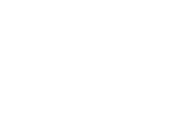This is just the standard Maxwell Boltzmann distribution, and Z is called the partition function (normalization constraint).

I do want to discuss a bit the assumptions that went into this derivation. The actual energy of our system is the Hamiltonian, but since an epistemic agent cannot know the actual state of the system, it must resort to averaging over all possible states to define the energy. If the actual system fluctuates a bit, this means that the actual energy is a time average of the energies the system fluctuates through. The statement that this temporal average is equivalent to the average over the ensemble of all possible states is known as the ergodic hypothesis. This is only possible because we assume the energy is constant (in the temporal average), which means that the system is in equilibrium. If the system was out of equilibrium, the ergodic hypothesis would no longer hold, and the epistemic agent would not have the belief distribution about the system derived above. Out of equilibrium dynamics are much more difficult to analyze, and we will not do so here.

The partition function turns out to be the most important quantity to analyze. Unfortunately it also happens to be notoriously difficult to calculate. Recalling the definition of the mean field, we can rewrite it as: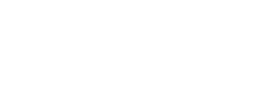This functional form is a sum over all possible magnetizations, followed by a sum over all states that have that magnetization. One thing we can instantly see from this is that the magnetic field, h, is acting as a bias. It suppresses belief in states that do not have a magnetization that is the same sign as the magnetic field. This is the reason why these quantities have the names they do: the magnetic field magnetizes the system.

With this machinery at hand we can now introduce dynamics. We initialize our system in any state we wish and then allow for transitions to new states: we let the system evolve in time. We use the probability of a graph state to find the probability that a single vertex transitions by simply holding all other vertices constant: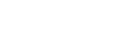At each timestep we pick some fraction of the vertices and calculate the probability that they change their state. With that probability we allow those vertices to either change or stay the same.

I’ve been running a lot of these types of simulations on scale free networks. Many networks in reality, including the Internet and Social Networks, have been shown to be scale free. I will write an upcoming post on how to actually create these types of networks, amongst others, but for this post I wanted to focus on the Ising model on graphs. Heres a simulation of all that I’ve been describing:

# Truthfulness and Perception in the 2016 PrimariesRecently I came by an article on Blue Nation Review claiming that Hillary Clinton is the most truthful of the candidates based on statistics from Politifact‘s Truth-O-Meter. Sure, she has the largest percentage of True statements, but a measure of truthfulness should draw on the full distribution of statements that a candidate has spoken, not just a particular subset. Clinton has also spoken partial truths, half truths, falsehoods, and racked up two cases of “Liar, liar, pants on fire!“. What other information is lurking in the full distributions that is lost in BNR’s simplistic analysis? Being enamored by information theory and epistemology, I decided to look at Clinton, Cruz, Kasich, Sanders, and Trump‘s Truth-o-Meter’s a little closer.

To understand this analysis, imagine an idealized thinking being, an epistemic agent(EA), which is initially completely ignorant of the truthfulness of candidates: the EA has no preference for believing what a candidate says when they say it. The EA is then exposed to statements by the candidates, and uses its fact checking facility (the journalists at Politifact) to inform it of the truthfulness of those statement. As it learns more its preferences shift. Analyzing more statements moves the EA from its tabula rasa starting state to a biased one where there is a preference to believe or not believe a candidate. The more statements the EA hears, the less the belief function changes, so that after enough statements, the EA has a pretty definite belief in the truthfulness of the candidates. We begin then with an ignorant EA, which opens up the Politifact site and begins reading the analyzed statements made by the candidates in the 2016 Primary election.Number of fact checks that have been done on each candidate by Politifact’s Truth-O-Meter Journalists.

The number of statements analyzed for each candidate varies(above). Kasich and Sanders have been the least scrutinized (61 and 75, respectively), while Hilary has been the most scrutinized (174 statements) by the site. Because of the differing amounts of data going into informing the EA, its belief in Clinton‘s truthfulness will be the least malleable, while in the case of Kasich it will be most malleable. A new datum from Clinton will not sway the EA much in terms of what it thinks of her as it would for both Kasich and Sanders. Cruz and Trump are in between these extremes. We call the EA’s initial beliefs about the candidates priors, and the belief’s after assimilating all the data on the candidate Truth-O-Meters the posteriors. You can read more about how epistemic states of knowledge change because of experiences here and here.

The posterior belief coming from an uninformative prior matches the normalized frequencies. Truth-O-Meter uses a six point scale, but in common speech truthfulness is a continuous variable due to its dependence on not just facts, but context, intensity of language, etc. The closer to 0, the more false a statement is; the closer to 1, the more true it is (recall that these terms are always with respect to the fact checking facility of the EA). Interpolation to the continuum belief distribution was accomplished by using a cubic Hermite spline, as described here. This choice does an excellent job preserving the shape of the discrete frequency distribution. Below we compare the discrete frequencies with the credence/belief distributions of the EA. The area under a curve represents the probability that a statement will have a particular range of truthfulness; the total area under any of these curves is unity.The frequency vs. truthfulness of the repertoire of statements said by each candidate.  Truthfulness of 0 corresponds to Truth-O-Meter’s “Liar, Liar, Pants on Fire” category, and 1 is “True.”The continuous credence(belief) function of an epistemic agent for each of the candidates. These are interpolated from the frequencies above by using a cubic spline.

These curves are interesting because they show what the beliefs of an epistemic agent look like under the assumption that it is judging the candidates solely on what they have said, and NOTHING else. Of course real people are not EAs, and past experiences end up coloring the judgements we make concerning new experiences. Nonetheless, an EA’s credence function might be a good indicator of what someone who is not very informed about the history of the candidates ends up thinking about them after reading through all the statements analyzed by Politifact.The cumulative distribution functions (cdf) derived from the credence functions for each candidate. The shaded region is to emphasize where the median lies for each of the candidates.Median truthfulness of each candidate. Clearly the candidates break up into two groups based on this measure.

What value of truthfulness does an EA associate with half the things that a candidate says? The answer is found by looking at the cumulative distribution functions (above). CDFs tell us how manifold statements are that come out of a candidates mouth below some value of truthfulness. We observe that the candidates cluster into groups: Clinton, Sanders, and Kasich lay in one, while Cruz and Trump occupy others. The truthfulness at which the EA believes that half of a candidate’s statements are more truthful than, and half are less truthful than, is the median.

Thus far, it is safe to say that both Trump and Cruz do not inspire much faith in the epistemic agent. The other three candidates all seem to be on equal footing, which means we need a better way to distinguish what the EA actually believes about them.

A natural next step would be to look at the moments of the credence distributions, however given the finite domain and presence of significant tails, standard interpretations of deviation, skewness, kurtosis,etc. would fail. Fortunately, rather than applying these ad hoc statistical measures, we can get a good grasp of the shape of the distributions by examining their Shannon entropy:Shannon entropy is an informational measure that quantifies the amount of uncertainty in a distribution. Credence distributions with low entropy contain a lot of information in them, meaning that an EA is less uncertain about the truthfulness of future statements. Our EA started off with beliefs that had maximal entropy: those based on ignorance. By interacting with the candidates, the EA has acquired information that has biased its beliefs so that it can make more informed judgements. Another way to say this is that the negentropy (the difference between maximal and actual entropy) is describing how an EA views the consistency of a candidate with regards to truthfulness.

There is a problem with this line of thinking, however: It only applies to median truthfulness values that are close to a half. The reason for this is that in order to get a high(or low) median truthfulness, the distribution must necessarily be skewed and hence have a lower entropy. One can estimate the maximum possible entropy of a distribution with truthfulness$\tau$ by considering a piecewise constant distribution. The resulting minimal negentropy can then be written in a closed analytical form:$-\log 2\sqrt{\tau(1-\tau)}$. The difference between the actual negentropy and this minimal value then gives a good measure of the consistency of a candidate in the eyes of the EA.

We plot both truthfulness (as measured by the median), versus consistency  (as measured by admissible negentropy), and see more of the differences between how an EA reasons about the candidates. Kasich falls out of the company of Clinton and Sanders; the EA thinks he’s nearly three times less consistent with his statements than Clinton. Cruz‘s consistency is on par with both Sanders and Clinton, while Trump beats everyone by a yuge margin in terms of how consistent he is about not being truthful. Sanders and Clinton appear grouped together, but the axes are a bit misleading. Sanders leads in truthfulness by about 3%, and in consistency by about 18% over Clinton.Consistency, as measured by entropic deviation from maximum, versus Truthfulness, as measured by the median of the continuum credence functions. Clustering is evident.

Using information theoretic tools, one can examine how completely rational epistemic agents construct their beliefs out of experiences. In this toy study we saw that the technique can be used to analyze how journalistic derived data concerning the factuality of primary candidates’ statements during a campaign can shed light on the truthfulness and consistency of those candidates. It also shows us that running with simple ad hoc statistics (cough cough) can lead to results that are no longer valid in a more detailed analysis. Speaking of more detail… there are a million ways (Information geometry of the candidate public perception manifold, time dependent Bayesian updating of EA states, testing the effects of nonuniform priors due to coupling between socioeconomic variables, etc.) to make this analysis more complete, but there’re only so many hours in a day. If you have any questions about my methods please feel free to leave a comment.